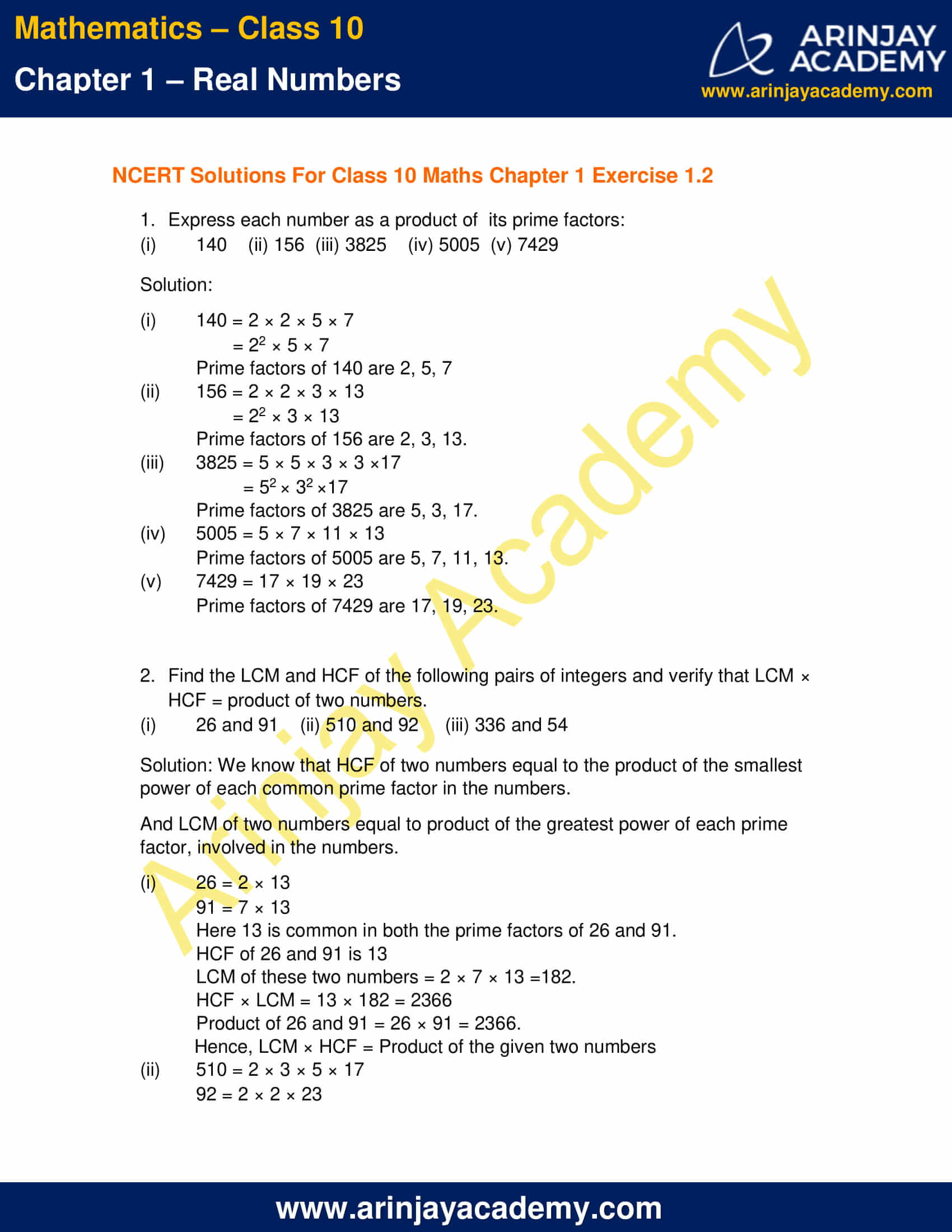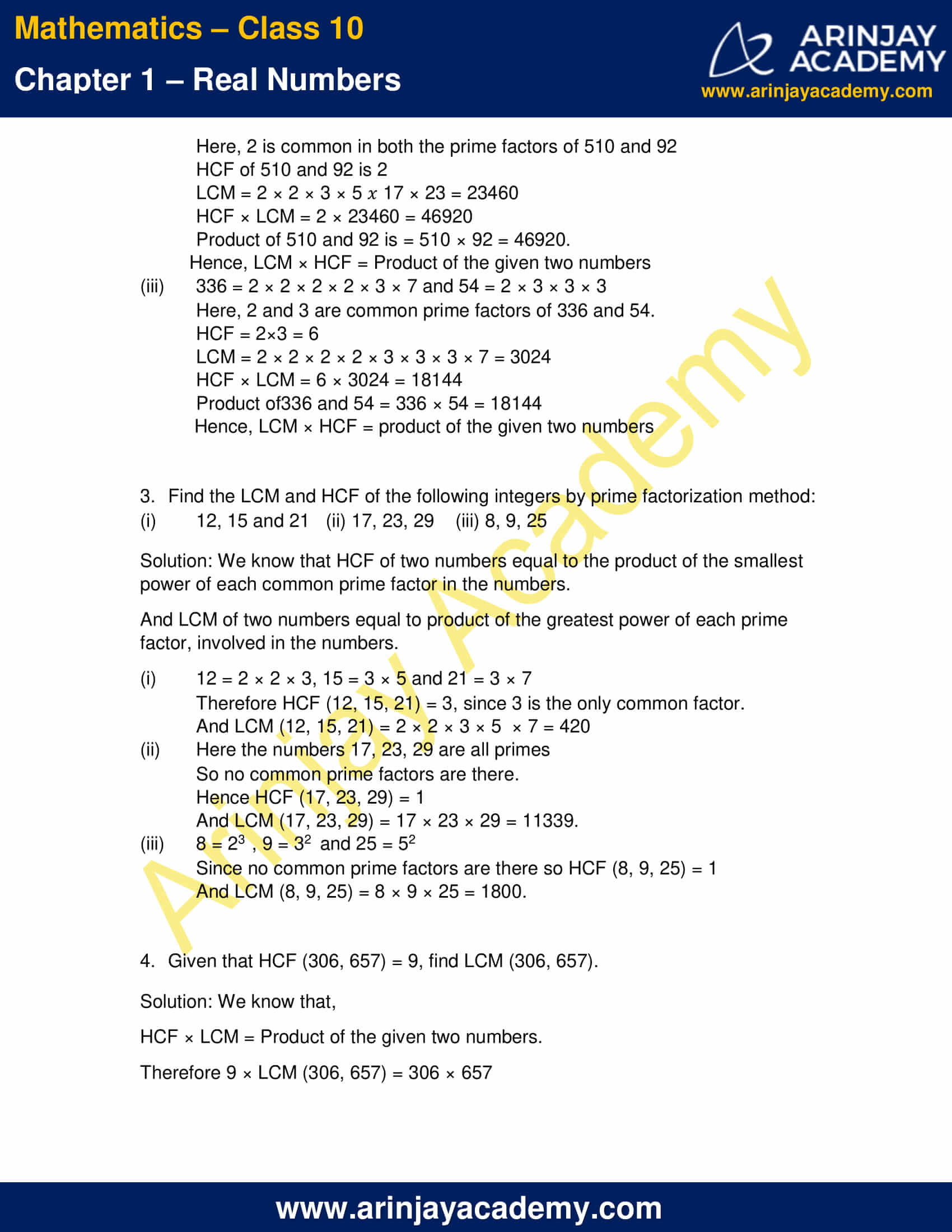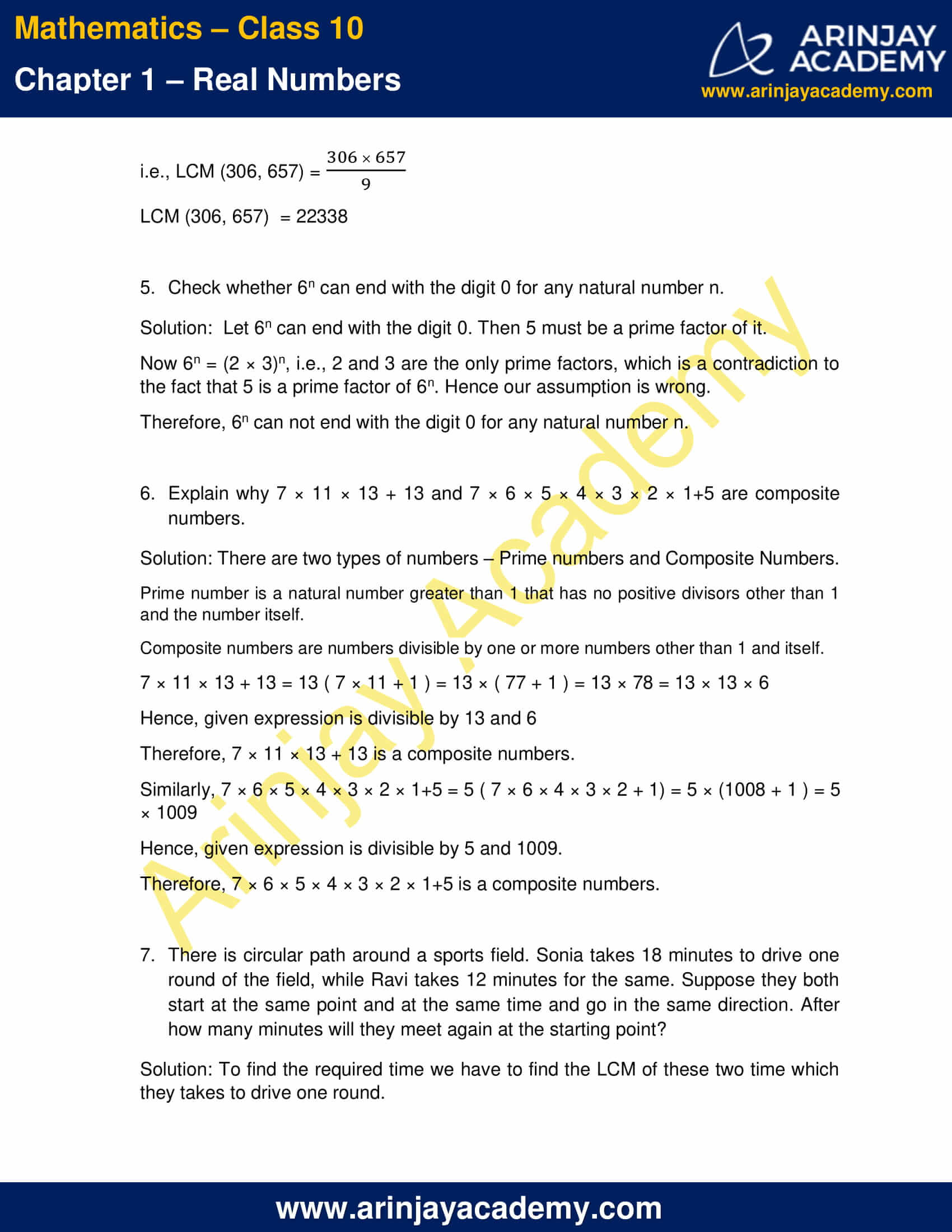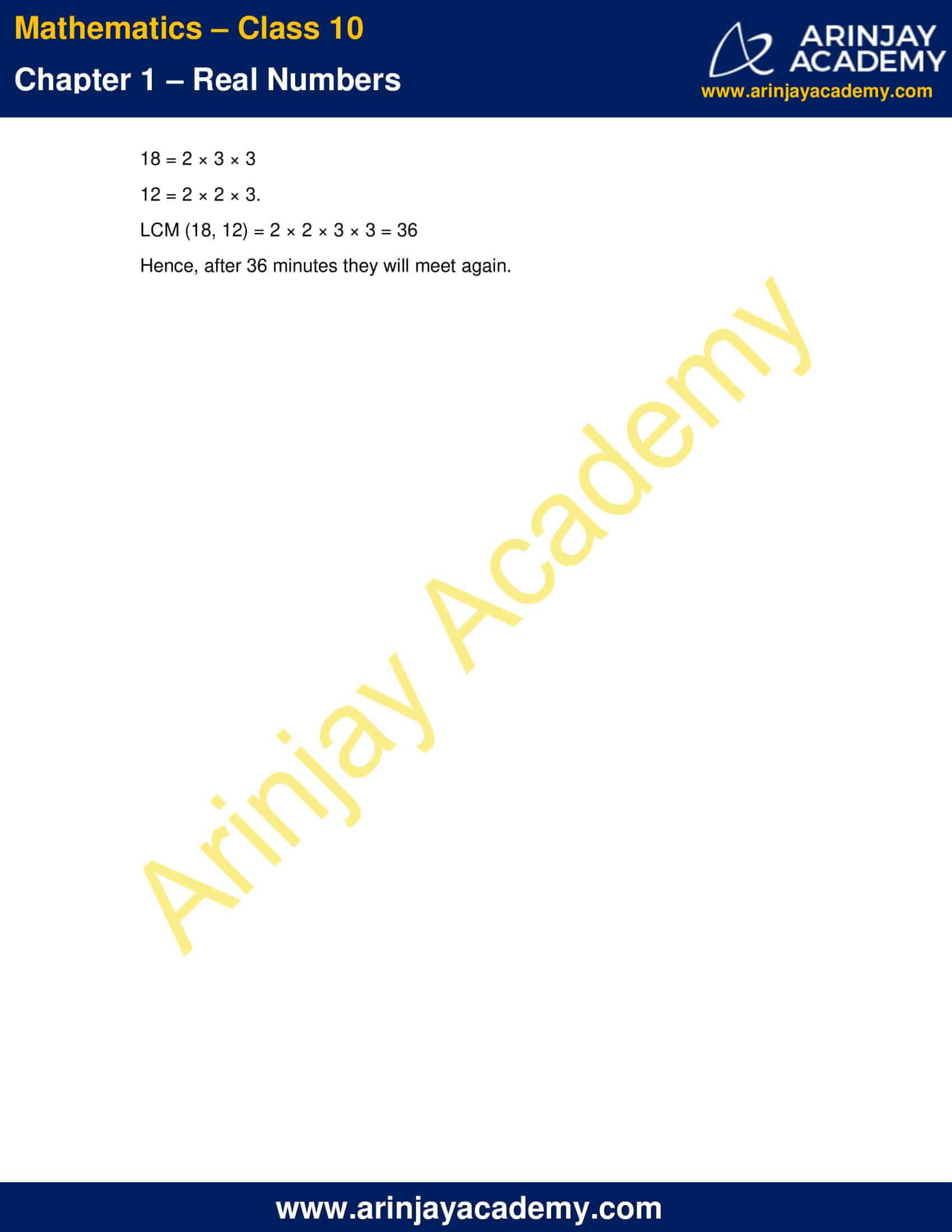# NCERT Solutions For Class 10 Maths Chapter 1 Exercise 1.2

Download NCERT Solutions For Class 10 Maths Chapter 1 Exercise 1.2 – Real Numbers. This Exercise contains 7 questions, for which detailed answers have been provided in this note. In case you are looking at studying the remaining Exercise for Class 10 for Maths NCERT solutions for other Chapters, you can click the link at the end of this Note.### NCERT Solutions For Class 10 Maths Chapter 1 Exercise 1.2 – Real NumbersNCERT Solutions For Class 10 Maths Chapter 1 Exercise 1.2 – Real Numbers

1. Express each number as a product of its prime factors:

(i) 140
(ii) 156
(iii) 3825
(iv) 5005
(v) 7429

Solution:

(i) 140 = 2 × 2 × 5 × 7
= 22 × 5 × 7
Prime factors of 140 are 2, 5, 7

(ii) 156 = 2 × 2 × 3 × 13
= 22 × 3 × 13
Prime factors of 156 are 2, 3, 13.

(iii) 3825 = 5 × 5 × 3 × 3 ×17
= 52 × 32 ×17
Prime factors of 3825 are 5, 3, 17.

(iv) 5005 = 5 × 7 × 11 × 13
Prime factors of 5005 are 5, 7, 11, 13.

(v) 7429 = 17 × 19 × 23
Prime factors of 7429 are 17, 19, 23.

2. Find the LCM and HCF of the following pairs of integers and verify that LCM × HCF = product of two numbers.

(i) 26 and 91
(ii) 510 and 92
(iii) 336 and 54

Solution:

We know that HCF of two numbers equal to the product of the smallest power of each common prime factor in the numbers.
And LCM of two numbers equal to product of the greatest power of each prime factor, involved in the numbers.

(i) 26 = 2 × 13
91 = 7 × 13
Here 13 is common in both the prime factors of 26 and 91.
HCF of 26 and 91 is 13
LCM of these two numbers = 2 × 7 × 13 =182.
HCF × LCM = 13 × 182 = 2366
Product of 26 and 91 = 26 × 91 = 2366.
Hence, LCM × HCF = Product of the given two numbers

(ii) 510 = 2 × 3 × 5 × 17
92 = 2 × 2 × 23
Here, 2 is common in both the prime factors of 510 and 92
HCF of 510 and 92 is 2
LCM = 2 × 2 × 3 × 5  17 × 23 = 23460
HCF × LCM = 2 × 23460 = 46920
Product of 510 and 92 is = 510 × 92 = 46920.
Hence, LCM × HCF = Product of the given two numbers

(iii) 336 = 2 × 2 × 2 × 2 × 3 × 7
54 = 2 × 3 × 3 × 3
Here, 2 and 3 are common prime factors of 336 and 54.
HCF = 2×3 = 6
LCM = 2 × 2 × 2 × 2 × 3 × 3 × 3 × 7 = 3024
HCF × LCM = 6 × 3024 = 18144
Product of336 and 54 = 336 × 54 = 18144
Hence, LCM × HCF = product of the given two numbers

3. Find the LCM and HCF of the following integers by prime factorization method:

(i) 12, 15 and 21
(ii) 17, 23, 29
(iii) 8, 9, 25

Solution: We know that HCF of two numbers equal to the product of the smallest power of each common prime factor in the numbers.
And LCM of two numbers equal to product of the greatest power of each prime factor, involved in the numbers.

(i) 12 = 2 × 2 × 3, 15 = 3 × 5 and 21 = 3 × 7
Therefore HCF (12, 15, 21) = 3, since 3 is the only common factor.
And LCM (12, 15, 21) = 2 × 2 × 3 × 5  × 7 = 420

(ii) Here the numbers 17, 23, 29 are all primes
So no common prime factors are there.
Hence HCF (17, 23, 29) = 1
And LCM (17, 23, 29) = 17 × 23 × 29 = 11339.

(iii) 8 = 23 , 9 = 32  and 25 = 52
Since no common prime factors are there so HCF (8, 9, 25) = 1
And LCM (8, 9, 25) = 8 × 9 × 25 = 1800.

4. Given that HCF (306, 657) = 9, find LCM (306, 657).

Solution:

We know that,
HCF × LCM = Product of the given two numbers.
Therefore 9 × LCM (306, 657) = 306 × 657
i.e., LCM (306, 657) = (306 x 657)/9
LCM (306, 657)  = 22338

5. Check whether 6n can end with the digit 0 for any natural number n.

Solution:

Let 6n can end with the digit 0. Then 5 must be a prime factor of it.
Now 6n = (2 × 3)n, i.e., 2 and 3 are the only prime factors, which is a contradiction to the fact that 5 is a prime factor of 6n. Hence our assumption is wrong.
Therefore, 6n can not end with the digit 0 for any natural number n.

6. Explain why 7 × 11 × 13 + 13 and 7 × 6 × 5 × 4 × 3 × 2 × 1+5 are composite numbers.

Solution:

There are two types of numbers – Prime numbers and Composite Numbers.
Prime number is a natural number greater than 1 that has no positive divisors other than 1 and the number itself.
Composite numbers are numbers divisible by one or more numbers other than 1 and itself.
7 × 11 × 13 + 13 = 13 ( 7 × 11 + 1 ) = 13 × ( 77 + 1 ) = 13 × 78 = 13 × 13 × 6
Hence, given expression is divisible by 13 and 6
Therefore, 7 × 11 × 13 + 13 is a composite numbers.
Similarly, 7 × 6 × 5 × 4 × 3 × 2 × 1+5 = 5 ( 7 × 6 × 4 × 3 × 2 + 1) = 5 × (1008 + 1 ) = 5 × 1009
Hence, given expression is divisible by 5 and 1009.
Therefore, 7 × 6 × 5 × 4 × 3 × 2 × 1+5 is a composite numbers.

7. There is circular path around a sports field. Sonia takes 18 minutes to drive one round of the field, while Ravi takes 12 minutes for the same. Suppose they both start at the same point and at the same time and go in the same direction. After how many minutes will they meet again at the starting point?

Solution:

To find the required time we have to find the LCM of these two time which they takes to drive one round.
18 = 2 × 3 × 3
12 = 2 × 2 × 3.
LCM (18, 12) = 2 × 2 × 3 × 3 = 36
Hence, after 36 minutes they will meet again.

NCERT Solutions for Class 10 Maths Chapter 1 Exercise 1.2 – Real Numbers, has been designed by the NCERT to test the knowledge of the student on the topic – The Fundamental Theorem of Arithmetic

### 1 thought on “NCERT Solutions For Class 10 Maths Chapter 1 Exercise 1.2”

1.Hi, very nice website

Reply